# Differential equation, partial, variational methods

Methods for solving boundary value problems for partial differential equations by reducing them (whenever possible) to suitably selected variational problems (i.e. to problems of finding the minimum or the maximum of a certain functional).

Variational methods are extensively used first of all in theoretical investigations (to demonstrate the theorems of existence, uniqueness and stability of solutions, to study the differentiability properties of solutions, in spectral theory, in the study of different optimization problems, etc.) and secondly in problems of finding approximate solutions of the equations. Approximate solutions of variational problems may be found by solving finite systems of algebraic equations; often algorithms for finding approximate solutions of variational problems are simpler and practically more convenient than the available algorithms for solving the same problems for partial differential equations. The variational method of studying boundary value problems was discovered in mid-19th century as the so-called Dirichlet principle of finding, in a domain, a harmonic function assuming on the boundarya given value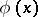,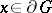, which, in the class of functions being considered, yields the minimum of the Dirichlet integral. Originally, the Dirichlet principle was used only in the theory of second-order linear elliptic equations (subsequently also in elliptic equations of higher order), after which it was applied in the theory of equations of other types, both linear and non-linear. The development of variational methods started from the work of B. Riemann, K. Weierstrass and D. Hilbert. Imbedding theorems and their generalizations played an important role in the development of variational methods, and in particular in their foundation.

A simple typical example of the application of variational methods is the solution of the Dirichlet problem for a second-order self-adjoint elliptic equation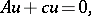(1)

where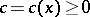,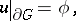(2)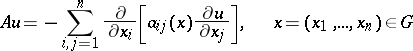(3)

(is a domain in a finite-dimensional Euclidean space,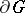is its boundary andis a function given on), and such that there exists a constant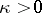such that for all points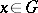and all numbers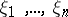the following inequality (ellipticity condition) is valid: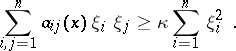In this case the variational method for solving the problem (1), (2) consists in finding a functionfor which the functional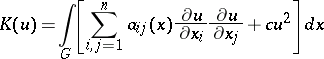assumes its lowest value in the class of admissible functions, i.e. of functions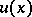for which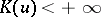and for which the boundary condition (2) is met (equation (1) is the Euler equation for the functional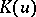). The variational method is applicable only if the class of admissible functions is non-empty. The conditions to be met by a functiondefined on the boundary so that the class of admissible functions is non-empty are given by the imbedding theorems. The functionfor which the functionalassumes its lowest value in the class of admissible functions is a generalized solution of the problem (1), (2) (cf. Differential equation, partial, functional methods) and is, for example in the classical case when the coefficientsof the operator (3) are continuously differentiable, an ordinary solution of the problem.

Another typical example of the use of variational methods is their application to finding eigen values and eigen functions of the operator (3).

A function giving the minimum of some functional may be obtained as the limit of a so-called minimizing sequence, i.e. a sequence of functions for which the values of the functional on these functions tend to the given minimum. Special methods (e.g. the Ritz method) have been developed for the construction of minimizing sequences and for the determination of their rate of convergence.